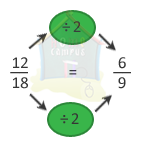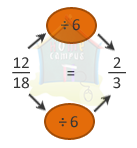## Equivalent Fractions

Practice Unlimited Questions

#### What are equivalent fractions?

Equivalent fractions are fractions that represents the same part of a whole. They have different numerators and denominators but with equal fractional values.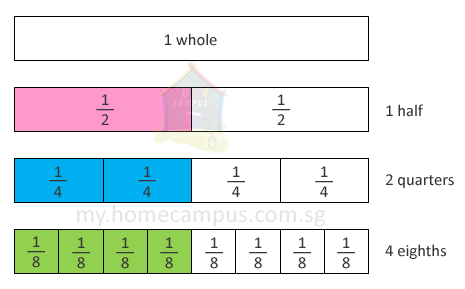1 out of 2 parts
=  2 out of 4 parts
=  4 out of 8 parts
Or,
 1 2

=

 2 4

=

 4 8

are equivalent fractions.

#### How to generate Equivalent Fractions?

Method 1: Multiply the numerator and the denominator by the same number.

Method 2: Divide the numerator and the denominator by the same number.

As long as you multiply or divide both numerator and denominator by the same number, the fractional value will not change.

#### 1. What are some of the equivalent fractions of 1 4 ?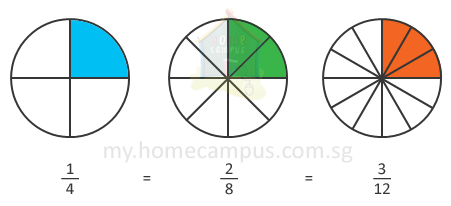Some of the equivalent fractions of
 1 4
are
 2 8
and
 3 12

#### 2. What are the first 3 equivalent fractions of 2 5 ?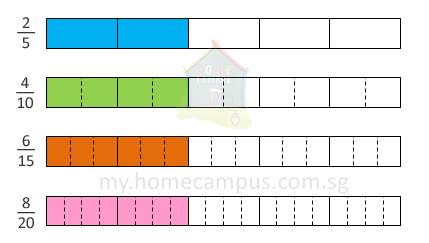A short-cut way to find an equivalent fraction is to multiply the numerator and denominator by the same number.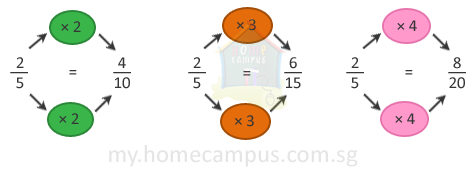2 5

=

 4 10

=

 6 15

=

 8 20
The first 3 equivalent fractions of
 2 5
are
 4 10
,
 6 15
and
 8 20

#### 3. Find the missing numbers in the equivalent fractions below: 12 18 = 6 ? , 12 18 = ? 3

Another way of finding equivalent fractions is to divide the numerator and denominator by the same number.